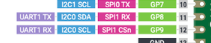# UART communication using arduino IDE by raspberry pi pico

hello
Currently, I am trying to UART communication with odrive V3.6 with raspberry pi pico, but I can not communicate. The pin of Raspberry pi pico is connected to TX (GP8) RX (GP9).The code looks like this:

``````#include <HardwareSerial.h>
//#include <SoftwareSerial.h>
#include <ODriveArduino.h>
// Printing with stream operator helper functions
template<class T> inline Print& operator <<(Print &obj,     T arg) {
obj.print(arg);
return obj;
}
template<>        inline Print& operator <<(Print &obj, float arg) {
obj.print(arg, 4);
return obj;
}

UART Serial2(8, 9);

HardwareSerial& odrive_serial = Serial2;

//odrive_serial = UART Serial2(8,9);

ODriveArduino odrive(odrive_serial);

void setup() {
// ODrive uses 115200 baud
odrive_serial.begin(115200);

// Serial to PC
Serial.begin(115200);
while (!Serial) ; // wait for Arduino Serial Monitor to open

Serial.println("ODriveArduino");
Serial.println("Setting parameters...");

// In this example we set the same parameters to both motors.
// You can of course set them different if you want.
// See the documentation or play around in odrivetool to see the available parameters
for (int axis = 0; axis < 2; ++axis) {
odrive_serial << "w axis" << axis << ".controller.config.vel_limit " << 10.0f << '\n';
odrive_serial << "w axis" << axis << ".motor.config.current_lim " << 11.0f << '\n';
// This ends up writing something like "w axis0.motor.config.current_lim 10.0\n"
}

Serial.println("Send the character '0' or '1' to calibrate respective motor (you must do this before you can command movement)");
Serial.println("Send the character 's' to exectue test move");
Serial.println("Send the character 'b' to read bus voltage");
Serial.println("Send the character 'p' to read motor positions in a 10s loop");
}

void loop() {

if (Serial.available()) {

// Run calibration sequence
if (c == '0' || c == '1') {
int motornum = c - '0';
int requested_state;

requested_state = ODriveArduino::AXIS_STATE_MOTOR_CALIBRATION;
Serial << "Axis" << c << ": Requesting state " << requested_state << '\n';
if (!odrive.run_state(motornum, requested_state, true)) return;

requested_state = ODriveArduino::AXIS_STATE_ENCODER_OFFSET_CALIBRATION;
Serial << "Axis" << c << ": Requesting state " << requested_state << '\n';
if (!odrive.run_state(motornum, requested_state, true, 25.0f)) return;

requested_state = ODriveArduino::AXIS_STATE_CLOSED_LOOP_CONTROL;
Serial << "Axis" << c << ": Requesting state " << requested_state << '\n';
if (!odrive.run_state(motornum, requested_state, false /*don't wait*/)) return;
}

// Sinusoidal test move
if (c == 's') {
Serial.println("Executing test move");
for (float ph = 0.0f; ph < 6.28318530718f; ph += 0.01f) {
float pos_m0 = 2.0f * cos(ph);
float pos_m1 = 2.0f * sin(ph);
odrive.SetPosition(0, pos_m0);
odrive.SetPosition(1, pos_m1);
delay(5);
}
}

if (c == 'b') {
odrive_serial << "r vbus_voltage\n";
Serial << "Vbus voltage: " << odrive.readFloat() << '\n';
}

// print motor positions in a 10s loop
if (c == 'p') {
static const unsigned long duration = 10000;
unsigned long start = millis();
while (millis() - start < duration) {
for (int motor = 0; motor < 2; ++motor) {
odrive_serial << "r axis" << motor << ".encoder.pos_estimate\n";